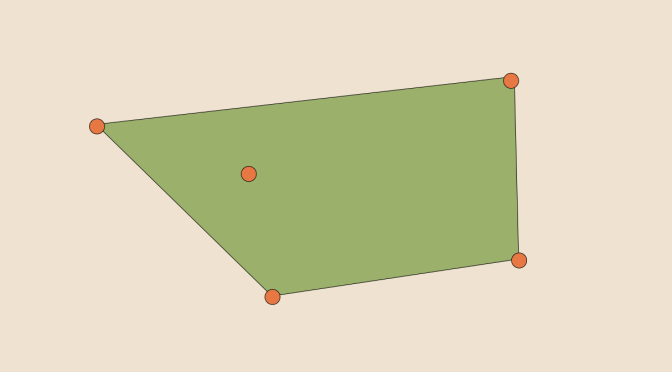A compact whose convex hull is not compact

We consider a topological vector space $$E$$ over the field of the reals $$\mathbb{R}$$. The convex hull of a subset $$X \subset E$$ is the smallest convex set that contains $$X$$.

The convex hull may also be defined as the intersection of all convex sets containing X or as the set of all convex combinations of points in X.

The convex hull of $$X$$ is written as $$\mbox{Conv}(X)$$.

Finite-dimensional case

We suppose in that paragraph that $$E=\mathbb{R}^n$$ is an $$n$$-dimensional real vector space. One can prove in that case that any convex combination of points of $$X \subset E$$ can be obtain be taking a convex combination of at most $$n+1$$ points of $$X$$. This is Carathéodory’s theorem:
$\mbox{Conv}(X)=\{\sum_{k=0}^n p_k x_k | \sum_{k=0}^n p_k = 1, p_k \ge 0, x_k \in X \mbox{ for } 0 \le k \le n \}$ An interesting consequence is that in the finite-dimensional case, the convex hull of a compact subset of $$\mathbb{R}^n$$ is compact. Indeed, consider a compact $$K \subset \mathbb{R}^n$$ and the map:
$\varphi : \left| \begin{array}{ll} K^{n+1} \times \Sigma_n & \longrightarrow \mathbb{R}^n\\ ((x_0, \dots, x_n),(p_0,\dots,p_n)) & \longmapsto \sum_{k=0}^n p_k x_k \end{array} \right.$ where $$\Sigma_n = \{(t_0, \dots, t_n) | t_k \ge 0 \mbox{ for } 0 \le k \le n \mbox{ and } t_0+ \cdots+t_n=1\}$$

$$\varphi$$ is continuous and $$K^{n+1} \times \Sigma_n$$ compact as a product of compacts. Therefore $$\mbox{Conv}(K)=\varphi(K^{n+1} \times \Sigma_n)$$ is also compact, as was required to be shown.

A counter-example in the infinite-dimensional case

Let’s take as the topological vector space $$E$$ the space $$\ell^2$$ of square summable sequences. The subset $$K$$ made up the sequence $$(\frac{e_n}{n})_{n \in \mathbb{N}^*}$$, where $$e_n(m)=1$$ if $$m = n$$ and $$e_n(m)=0$$ if $$m \neq n$$, and its null limit is compact.

The sequence $$u=(0,\frac{1}{2} \frac{1}{1}, \frac{1}{2^2} \frac{1}{2}, \dots,\frac{1}{2^n} \frac{1}{n},\dots)$$ belongs to $$\ell^2$$. Moreover:
$u=\lim\limits_{n \rightarrow \infty} \frac{\sum_{i=1}^n \frac{1}{2^i} \frac{e_i}{i}}{\sum_{i=1}^n \frac{1}{2^i}}$
So $$u$$ belongs to the closure of $$\mbox{Conv}(K)$$. Therefore, if $$\mbox{Conv}(K)$$ was compact, $$\mbox{Conv}(K)$$ will be closed and $$u$$ will belong to $$\mbox{Conv}(K)$$ which is not the case. Conclusion: $$\mbox{Conv}(K)$$ is not compact.